# Comparison of Isolated DC-DC Converter Topologies for High Power Applications

Both of these topologies can operate at high voltage and high power applications and have very low switching and conductive losses. Therefore, PSFB and FBLLC are the most widely used isolated DC-DC converters in a range of 500W to 50kW, which covers a number of different industries and applications, including electric vehicle chargers.

*Note: Full design of converters is outside the scope of this application note.

## Phase Shifted Full Bridge (PSFB)

• Fixed frequency, hence easier synchronization
• The output voltage is controlled by changing the phase shift between Q1 and Q4.
• Wide range of output voltage without compromising the efficiency
• Buck derived topology, the output voltage can be easily derived from the transformer turns ratio and the phase shift.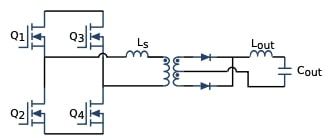## Full Bridge LLC (FB-LLC)

• The output voltage is controlled changing the operating frequency. Synchronization is more difficult due to changing frequency.
• The highest efficiency when at resonance (Higher than PSFB), and lower EMI noise.
• Designed using the first harmonic approximation, which becomes inaccurate away from the resonance.
• Operated ar burst mode at light load to avoid increasing frequency inadequately.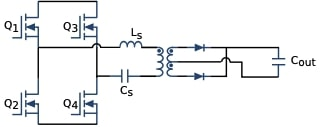### Design of the PSFB magnetic componentsThe transformer needs to be designed for the required turns ratio and minimum magnetizing inductance for current-mode control.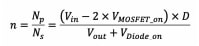Series inductance needs to be designed for ZVS, such that it can store the energy from MOSFET capacitors.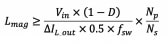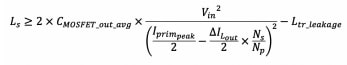### Design of the FB-LLC magnetic components

• The transformer turns ratio is chosen to fit the output to the input voltage ratio.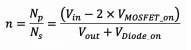• Based on the operating requirements (range) of the FB-LLC, the resonant tank's quality factor Qe and the ratio Ln between the magnetizing inductance of the transformer and series inductance needs to be chosen.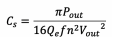• Based on Qe, the series capacitance of the resonant tank is chosen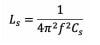• Series inductance is designed for a chosen resonant frequency and the capacitance value •Magnetizing inductance of the transformer is calculated from chosen Ln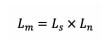• The magnetizing inductance of the transformer is calculated from chosen Ln

#### CONCLUSIONS

Full Bridge LLC is the most efficient solution for point of load converter application, and also has less EMI noise. However, for easier design and more flexible and robust systems, Phase Shifted Full Bridge is a better option. Frenetic can design and prototype both of these converters, along with magnetic elements. Moreover, Frenetic's artificial intelligence can merge the transformer and the series inductance into one magnetic component.

Do you want the full version?